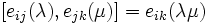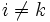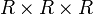# Steinberg group over a unital ring

## Definition

Suppose$R$ is a (associative) unital ring and$n$ is a natural number. The Steinberg group of degree$n$ over$R$ (also called the unstable Steinberg group), denoted$\operatorname{St}_n(R)$ or$\operatorname{St}(n,R)$, is defined by the following presentation:

• The generating set is as follows: For every element$\lambda \in R$ and for$1 \le i,j \le n$,$i \ne j$, we have a generator$e_{ij}(\lambda)$.
• The relations are as follows. In all cases,$\lambda,\mu$ vary freely over all of$R$, and are allowed to be equal or distinct.
Relation type Count of such relations (combinatorial description) Comments$e_{ij}(\lambda)e_{ij}(\mu) = e_{ij}(\lambda + \mu)$$n(n - 1)$ copies of$R \times R$ This implies that$e_{ij}(0)$ is the identity element.$[e_{ij}(\lambda),e_{jk}(\mu)] = e_{ik}(\lambda \mu)$ for$i \ne k$$n(n-1)(n-2)$ copies of$R \times R \times R$ The$[ \ , \ ]$ denotes the group commutator operation. It does not matter whether we use the left or right normed convention for the commutator (though this becomes clear only after looking at the entire presentation).$[e_{ij}(\lambda),e_{kl}(\mu)] = 1$ (i.e., is the identity element) for$i \ne l, j \ne k$.$n(n-1)^2(n - 2)$ copes of$R \times R$ (note: we can half this number by noting that one commutator being trivial implies the same commutator in reverse order is also trivial)

### Case$n= 1$

The case$n = 1$ gives a trivial group because there are no generators and no relations. This is not of interest.

### Case$n = 2$

The case$n = 2$ is somewhat different from the case$n \ge 3$. For$n = 2$, we simply get a free product of two copies of the additive group of$R$. This is because there are no relations of the commutator type, and hence, there are no relations connecting the$e_{12}(\lambda)$ with the$e_{21}(\lambda)$ type generators.

There is an alternative definition of Steinberg group some people use for$n = 2$ that is intended to remedy this problem. What is it?

### Stable version

The stable Steinberg group over a unital ring is similar to the above except that we have no size restrictions on$i$ and$j$.

## Facts

For every$R$ and$n$, there is a standard homomorphism from the Steinberg group to the group generated by elementary matrices over a unital ring$E_n(R)$. This homomorphism sends the generator$e_{ij}(\lambda)$ to the elementary matrix$e_{ij}(\lambda)$, i.e., the matrix with$1$s on the diagonal,$\lambda$ in the$(ij)^{th}$ entry, and$0$s elsewhere. When$R$ is a field, the group$E_n(R)$ coincides with the special linear group$SL_n(R)$ (see Elementary matrices of the first kind generate the special linear group over a field).

Note that$E_n(R)$ coinciding with$SL_n(R)$ also holds when$R$ is a Euclidean domain.